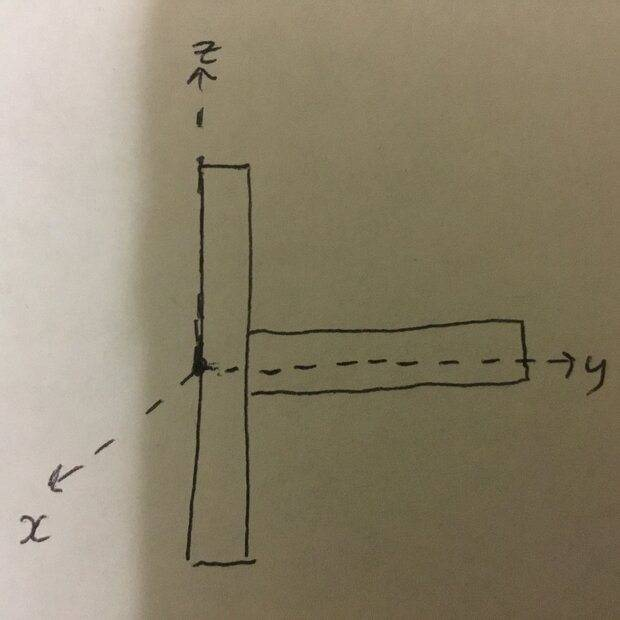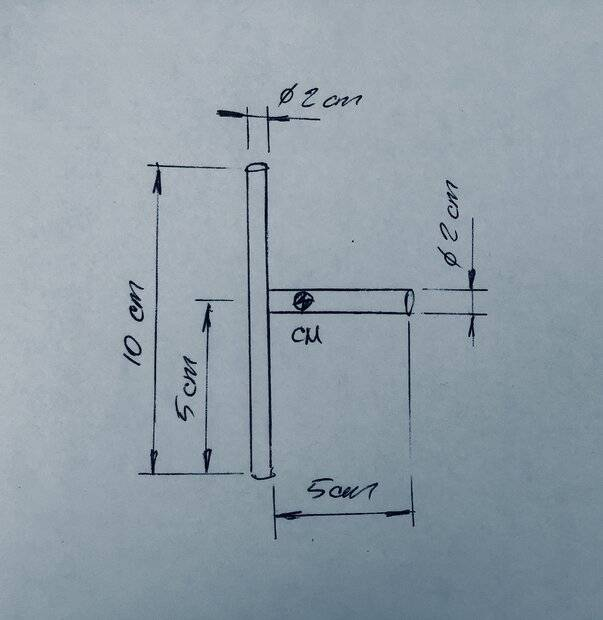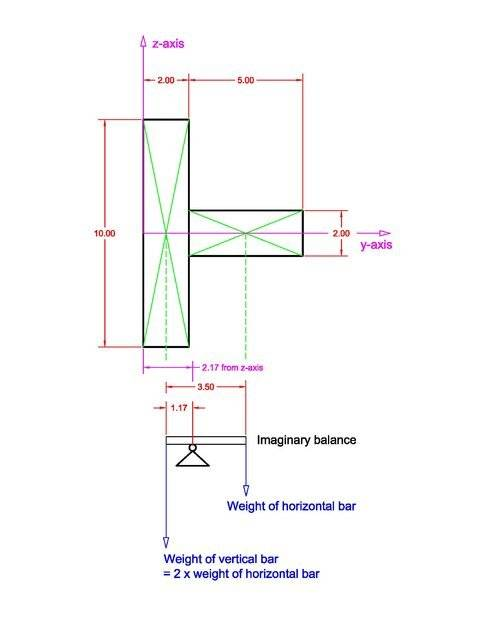# Moment of inertia of T bar about 3 axes

so_gr_lo
Homework Statement:
A bar of length 10cm and diameter 2cm is positioned vertically, it has another bar of length 5cm and diameter 2cm attached at a right angle to its middle. The density is 800 kg m^-3. Find the position of the centre of mass. Then find the moments of inertia about 3 perpendicular axis through its centre of mass.
Relevant Equations:
Xcm = m1x1 + m2x2 / m1 + m2

I = mr^2/2 (for a bar)
Using the equation above I get Xcm = 0.022 m. I set the origin be at the left of the vertical rod parallel to its centre of mass as in the diagram. But I’m not sure if the equation is correct for 3d.for the moments of inertia I am using

I = Icm + md^2
= (mr^2)/2 + md^2

where d is the distance from the centre of mass to the axis. Does this equation seem correct?

Gold Member
I = Icm + md^2
= (mr^2)/2 + md^2

where d is the distance from the centre of mass to the axis. Does this equation seem correct?
I don't believe so. Please elaborate on your calculations. Also, figure out how to format your mathematics using Latex so it is easily read.

##\bar{x}## looks good.

See LaTeX Guide

Last edited:
•topsquark
Homework Helper
Gold Member
2022 Award
I = mr^2/2 (for a bar)

•topsquark
Gold Member
Aha! good point.

so_gr_lo
thats the result for a cylinder about its symmetry axis, not sure if that’s the axis I’m supposed to use

•malawi_glenn
Gold Member
thats the result for a cylinder about its symmetry axis, not sure if that’s the axis I’m supposed to use
It's not a "which one" its multiple.

•topsquark
Homework Helper
Gold Member
2022 Award
thats the result for a cylinder about its symmetry axis, not sure if that’s the axis I’m supposed to use
I assume you mean its axis of rotational symmetry. There are also two axes of reflective symmetry normal to that one and to each other.
If the rotation is about the axis of rotational symmetry for one, which of its axes is the other rotating about?

•erobz and topsquark
Homework Helper
Gold Member
The diagram shows one axis not crossing the center of mass.
Locating that center should be the first step.

Homework Helper
Gold Member
2022 Award
The diagram shows one axis not crossing the center of mass.
Locating that center should be the first step.
The question specifically asks for the centre of mass and the OP found it correctly in post #1.
The axes the solver chooses for the purpose of analysis need not be those about which the MoI is requested.

Homework Helper
Gold Member
The question specifically asks for the centre of mass and the OP found it correctly in post #1.
The axes the solver chooses for the purpose of analysis need not be those about which the MoI is requested.
It seems to me that the shown result Xcm = 0.022 m in post #1 is not numerically correct.
Also, according to the shown disposition of the axes in the diagram, the center of mass should not be spatially located on the x axis.

Could you please, explain your following statement a little more for me?:
“There are also two axes of reflective symmetry normal to that one and to each other.“
Sorry, I can only see one.

Gold Member
It seems to me that the shown result Xcm = 0.022 m in post #1 is not numerically correct.
Also, according to the shown disposition of the axes in the diagram, the center of mass should not be spatially located on the x axis.

Could you please, explain your following statement a little more for me?:
“There are also two axes of reflective symmetry normal to that one and to each other.“
Sorry, I can only see one.
Oh I see. ##x_{cm}## is not the proper label. Per the diagram they found ##y_{cm}##. I think we are assuming ##x_{cm} = 0 = z_{cm}##.

Homework Helper
Gold Member
Oh I see. ##x_{cm}## is not the proper label. Per the diagram they found ##y_{cm}##. I think we are assuming ##x_{cm} = 0 = z_{cm}##.
Yes.
I may be wrong, but I am getting ##y=1.83~cm## as the location for CM measured from the left surface of the 10 cm vertical rod (plane x-z).

Gold Member
Yes.
I may be wrong, but I am getting ##y=1.83~cm## as the location for CM measured from the left surface of the 10 cm vertical rod (plane x-z).
Do you mean ##18.3 \rm{cm}##? I got ## y_{cm} = 21.7 \rm{cm}## How are you assuming they are connected ( I'm assuming they are basically set next to each other and welded)?

Homework Helper
Gold Member
2022 Award
Could you please, explain your following statement a little more for me?:
“There are also two axes of reflective symmetry normal to that one and to each other.“
I should have written "a plane of rotational symmetry and two planes of reflective symmetry ".
It seems to me that the shown result Xcm = 0.022 m in post #1
I had not noticed @so_gr_lo's confusion between the x and y axes.

•Lnewqban
Homework Helper
Gold Member
2022 Award
Do you mean ##18.3 \rm{cm}##? I got ## y_{cm} = 21.7 \rm{cm}## How are you assuming they are connected ( I'm assuming they are basically set next to each other and welded)?
It is not entirely clear whether the cross section is circular or square. It says "diameter", but I would have called them rods if circular; bar suggests square. And welding them together would be tricky if rods.

Homework Helper
Gold Member
Do you mean ##18.3 \rm{cm}##? I got ## y_{cm} = 21.7 \rm{cm}## How are you assuming they are connected ( I'm assuming they are basically set next to each other and welded)?
As I understand it (again, perhaps wrongly), the biggest dimensions of the assembled “T” are 7 cm and 10 cm.Last edited:
•erobz
Gold Member
It is not entirely clear whether the cross section is circular or square. It says "diameter", but I would have called them rods if circular; bar suggests square. And welding them together would be tricky if rods.
I assume circular rods (as in cylinders).

And welding them together would be tricky if rods

I worked in a steel mill... believe me when I say...It can be done quite easily!

I think another interpretation is that this is a solid formed piece where the end of the horizontal rod is inserted into the vertical rod up to its midpoint.

•Lnewqban
Gold Member
As I understand it (again, perhaps wrongly), the biggest dimensions of the assembled “T” are 7 cm and 10 cm.
No, that's how I assumed it too. Maybe I made a computational mistake.

Homework Helper
Gold Member
No, that's how I assumed it too. Maybe I made a computational mistake.
The mass of the vertical rod is two times the mass of the horizontal one.

Gold Member
So, ignoring the weld. Here is what I did:

$$y_{cm} = \frac{\rho \frac{\pi D^2}{4} \cdot 10 \cdot 1 + \rho \frac{\pi D^2}{4} \cdot 5 \cdot ( 2 + 2.5) }{ \rho \frac{\pi D^2}{4} ( 10 + 5 ) } = \frac{10+5\cdot(2+2.5)}{15} \approx 2.17 \rm{cm}$$

I did make a mistake. It was ##2.17 \rm{cm}## not ##21.7 \rm{cm}##. We still get different results as you are getting ##1.83 \rm{cm}## ?

Last edited:
•Lnewqban
Homework Helper
Gold Member
I should have written "a plane of rotational symmetry and two planes of reflective symmetry ".
Thank you much, @haruspex

Homework Helper
Gold Member
So, ignoring the weld. Here is what I did:

$$y_{cm} = \frac{\rho \frac{\pi D^2}{4} \cdot 10 \cdot 1 + \rho \frac{\pi D^2}{4} \cdot 5 \cdot ( 2 + 2.5) }{ \rho \frac{\pi D^2}{4} ( 10 + 5 ) } = \frac{10+5\cdot(2+2.5)}{15} \approx 2.17 \rm{cm}$$

I did make a mistake. It was ##2.17 \rm{cm}## not ##21.7 \rm{cm}##. We still get different results as you are getting ##1.83 \rm{cm}## ?
Your calculation is correct; mine was not.
Please, see attached scaled diagram (PDF format below).
Thank you very much, @erobz#### Attachments

• Bars COM.pdf
26.3 KB · Views: 12
•erobz